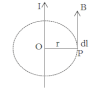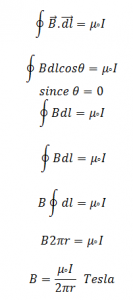# Magnetic Field Of Current Carrying ConductorConsider a straight conductor carrying current I is as shown in figure. Let P be the point at a distance r from conductor where we have to draw a concentric circle of radius r. The direction of magnetic field at pint P is given by the tangent of the circle. Where the direction of B and dl is along the same direction and angle between them is 0°.

From Ampere’s Law we know the magnetic field due to straight current carrying conductor is:This is the magnetic field due to straight current carrying conductor.